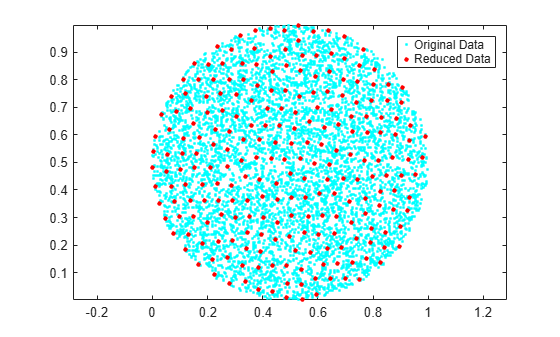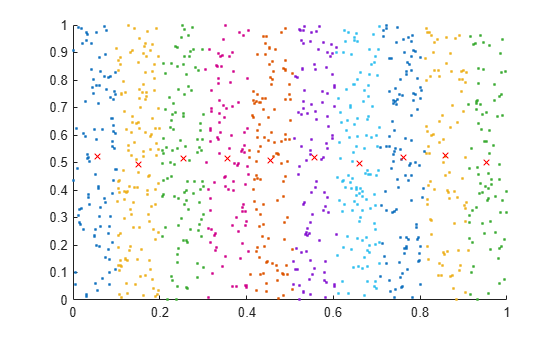# uniquetol

Unique values within tolerance

## Syntax

``C = uniquetol(A,tol)``
``C = uniquetol(A)``
``````[C,IA,IC] = uniquetol(___)``````
``[___] = uniquetol(___,Name,Value)``

## Description

example

````C = uniquetol(A,tol)` returns the unique elements in `A` using tolerance `tol`. Two values, `u` and `v`, are within tolerance if`abs(u-v) <= tol*max(abs(A(:)))`That is, `uniquetol` scales the `tol` input based on the magnitude of the data.`uniquetol` is similar to `unique`. Whereas `unique` performs exact comparisons, `uniquetol` performs comparisons using a tolerance.```

example

````C = uniquetol(A)` uses a default tolerance of `1e-6` for single-precision inputs and `1e-12` for double-precision inputs.```

example

``````[C,IA,IC] = uniquetol(___)``` returns index vectors `IA` and `IC`, such that `C = A(IA)` and `A~C(IC)` (or `A(:)~C(IC)` if `A` is a matrix), where `~` means the values are within tolerance of each other. You can use any of the input arguments in previous syntaxes.```

example

````[___] = uniquetol(___,Name,Value)` uses additional options specified by one or more Name-Value pair arguments using any of the input or output argument combinations in previous syntaxes. For example, `uniquetol(A,'ByRows',true)` determines the unique rows in `A`.```

## Examples

collapse all

Create a vector `x`. Obtain a second vector `y` by transforming and untransforming `x`. This transformation introduces round-off differences in `y`.

```x = (1:6)'*pi; y = 10.^log10(x);```

Verify that `x` and `y` are not identical by taking the difference.

`x-y`
```ans = 6×1 10-14 × 0.0444 0 0 0 0 -0.3553 ```

Use `unique` to find the unique elements in the concatenated vector `[x;y]`. The `unique` function performs exact comparisons and determines that some values in `x` are not exactly equal to values in `y`. These are the same elements that have a nonzero difference in `x-y`. Thus, `c` contains values that appear to be duplicates.

`c = unique([x;y])`
```c = 8×1 3.1416 3.1416 6.2832 9.4248 12.5664 15.7080 18.8496 18.8496 ```

Use `uniquetol` to perform the comparison using a small tolerance. `uniquetol` treats elements that are within tolerance as equal.

`C = uniquetol([x;y])`
```C = 6×1 3.1416 6.2832 9.4248 12.5664 15.7080 18.8496 ```

By default, `uniquetol` looks for unique elements that are within tolerance, but it also can find unique rows of a matrix that are within tolerance.

Create a numeric matrix, `A`. Obtain a second matrix, `B`, by transforming and untransforming `A`. This transformation introduces round-off differences to `B`.

```A = [0.05 0.11 0.18; 0.18 0.21 0.29; 0.34 0.36 0.41; 0.46 0.52 0.76]; B = log10(10.^A);```

Use `unique` to find the unique rows in `A` and `B`. The `unique` function performs exact comparisons and determines that all of the rows in the concatenated matrix `[A;B]` are unique, even though some of the rows differ by only a small amount.

`unique([A;B],'rows')`
```ans = 8×3 0.0500 0.1100 0.1800 0.0500 0.1100 0.1800 0.1800 0.2100 0.2900 0.1800 0.2100 0.2900 0.3400 0.3600 0.4100 0.3400 0.3600 0.4100 0.4600 0.5200 0.7600 0.4600 0.5200 0.7600 ```

Use `uniquetol` to find the unique rows. `uniquetol` treats rows that are within tolerance as equal.

`uniquetol([A;B],'ByRows',true)`
```ans = 4×3 0.0500 0.1100 0.1800 0.1800 0.2100 0.2900 0.3400 0.3600 0.4100 0.4600 0.5200 0.7600 ```

Create a vector, `x`. Obtain a second vector, `y`, by transforming and untransforming `x`. This transformation introduces round-off differences to some elements in `y`.

```x = (1:5)'*pi; y = 10.^log10(x);```

Combine `x` and `y` into a single vector, `A`. Use `uniquetol` to reconstruct `A`, treating the values that are within tolerance as equal.

`A = [x;y]`
```A = 10×1 3.1416 6.2832 9.4248 12.5664 15.7080 3.1416 6.2832 9.4248 12.5664 15.7080 ```
```[C,IA,IC] = uniquetol(A); newA = C(IC)```
```newA = 10×1 3.1416 6.2832 9.4248 12.5664 15.7080 3.1416 6.2832 9.4248 12.5664 15.7080 ```

You can use `newA` with `==` or functions that use exact equality like `isequal` or `unique` in subsequent code.

`D1 = unique(A)`
```D1 = 6×1 3.1416 3.1416 6.2832 9.4248 12.5664 15.7080 ```
`D2 = unique(newA)`
```D2 = 5×1 3.1416 6.2832 9.4248 12.5664 15.7080 ```

Create a cloud of 2-D sample points constrained to be inside a circle of radius `0.5` centered at the point $\left(\frac{1}{2},\frac{1}{2}\right)$.

```x = rand(10000,2); insideCircle = sqrt((x(:,1)-.5).^2+(x(:,2)-.5).^2)<0.5; y = x(insideCircle,:);```

Find a reduced set of points, such that each point of the original dataset is within tolerance of a point.

```tol = 0.05; C = uniquetol(y,tol,'ByRows',true);```

Plot the reduced set of points as red dots on top of the original data set. The red dots are all members of the original data set. All the red dots are at least a distance `tol` apart.

```plot(y(:,1),y(:,2),'.') hold on axis equal plot(C(:,1), C(:,2), '.r', 'MarkerSize', 10)```Create a vector of random numbers and determine the unique elements using a tolerance. Specify `OutputAllIndices` as `true` to return all of the indices for the elements that are within tolerance of the unique values.

```A = rand(100,1); [C,IA] = uniquetol(A,1e-2,'OutputAllIndices',true);```

Find the average value of the elements that are within tolerance of the value `C(2)`.

`C(2)`
```ans = 0.0318 ```
`allA = A(IA{2})`
```allA = 3×1 0.0357 0.0318 0.0344 ```
`aveA = mean(allA)`
```aveA = 0.0340 ```

By default, `uniquetol` uses a tolerance test of the form `abs(u-v) <= tol*DS`, where `DS` automatically scales based on the magnitude of the input data. You can specify a different `DS` value to use with the `DataScale` option. However, absolute tolerances (where `DS` is a scalar) do not scale based on the magnitude of the input data.

First, compare two small values that are a distance `eps` apart. Specify `tol` and `DS` to make the within tolerance equation: `abs(u-v) <= 10^-6`.

```x = 0.1; uniquetol([x, exp(log(x))], 10^-6, 'DataScale', 1)```
```ans = 0.1000 ```

Next, increase the magnitude of the values. The round-off error in the calculation `exp(log(x))` is proportional to the magnitude of the values, specifically to `eps(x)`. Even though the two large values are a distance `eps` from one another, `eps(x)` is now much larger. Therefore, `10^-6` is no longer a suitable tolerance.

```x = 10^10; uniquetol([x, exp(log(x))], 10^-6, 'DataScale', 1)```
```ans = 1×2 1010 × 1.0000 1.0000 ```

Correct this issue by using the default (scaled) value of `DS`.

```format long Y = [0.1 10^10]; uniquetol([Y, exp(log(Y))])```
```ans = 1×2 1010 × 0.000000000010000 1.000000000000000 ```

Create a set of random 2-D points, then use `uniquetol` to group the points into vertical bands that have a similar (within tolerance) x-coordinate. Use these options with `uniquetol`:

• Specify `ByRows` as `true` since the point coordinates are in the rows of `A`.

• Specify `OutputAllIndices` as `true` to return the indices for all points that have an x-coordinate within tolerance of each other.

• Specify `DataScale` as `[1 Inf]` to use an absolute tolerance for the `x`-coordinate while ignoring the `y`-coordinate.

```A = rand(1000,2); DS = [1 Inf]; [C,IA] = uniquetol(A, 0.1, 'ByRows', true, ... 'OutputAllIndices', true, 'DataScale', DS);```

Plot the points and average value for each band.

```hold on for k = 1:length(IA) plot(A(IA{k},1), A(IA{k},2), '.') meanAi = mean(A(IA{k},:)); plot(meanAi(1), meanAi(2), 'xr') end```## Input Arguments

collapse all

Query array, specified as a scalar, vector, matrix, or multidimensional array. `A` must be full.

Data Types: `single` | `double`

Comparison tolerance, specified as a positive, real scalar. `uniquetol` scales the `tol` input using the maximum absolute value in input array `A`. Then `uniquetol` uses the resulting scaled comparison tolerance to determine which elements in `A` are unique. If two elements in `A` are within tolerance of each other, then `uniquetol` considers them to be equal.

Two values, `u` and `v`, are within tolerance if `abs(u-v) <= tol*max(abs(A))`.

To specify an absolute tolerance, specify both `tol` and the `'DataScale'` Name-Value pair.

Example: `tol = 0.05`

Example: ```tol = 1e-8```

Example: `tol = eps`

Data Types: `single` | `double`

### Name-Value Pair Arguments

Specify optional comma-separated pairs of `Name,Value` arguments. `Name` is the argument name and `Value` is the corresponding value. `Name` must appear inside quotes. You can specify several name and value pair arguments in any order as `Name1,Value1,...,NameN,ValueN`.

Example: `C = uniquetol(A,'ByRows',true)`

Output index type, specified as the comma-separated pair consisting of `'OutputAllIndices'` and either `false` (default), `true`, `0`, or `1`. `uniquetol` interprets numeric `0` as `false` and numeric `1` as `true`.

When `OutputAllIndices` is `true`, the `uniquetol` function returns the second output, `IA`, as a cell array. The cell array contains the indices for all elements in `A` that are within tolerance of a value in `C`. That is, each cell in `IA` corresponds to a value in `C`, and the values in each cell correspond to locations in `A`.

Example: `[C,IA] = uniquetol(A,tol,'OutputAllIndices',true)`

Row comparison toggle, specified as the comma-separated pair consisting of `'ByRows'` and either `false` (default), `true`, `0`, or `1`. `uniquetol` interprets numeric `0` as `false` and numeric `1` as `true`. Use this option to find rows in `A` that are unique, within tolerance.

When `ByRows` is `true`:

• `A` must be a 2-D array.

• `uniquetol` compares the rows of `A` by considering each column separately. For two rows to be within tolerance of one another, each column has to be in tolerance.

• Each row in `A` is within tolerance of a row in `C`. However, no two rows in `C` are within tolerance of each other.

Two rows, `u` and `v`, are within tolerance if `all(abs(u-v) <= tol*max(abs(A),[],1))`.

Example: `C = uniquetol(A,tol,'ByRows',true)`

Scale of data, specified as the comma-separated pair consisting of `'DataScale'` and either a scalar or vector. Specify `DataScale` as a numeric scalar, `DS`, to change the tolerance test to be `abs(u-v) <= tol*DS`.

When used together with the `ByRows` option, the `DataScale` value also can be a vector. In this case, each element of the vector specifies `DS` for a corresponding column in `A`. If a value in the `DataScale` vector is `Inf`, then `uniquetol` ignores the corresponding column in `A`.

Example: `C = uniquetol(A,'DataScale',1)`

Example: ```[C,IA,IC] = uniquetol(A,'ByRows',true,'DataScale',[eps(1) eps(10) eps(100)])```

Data Types: `single` | `double`

## Output Arguments

collapse all

Unique elements in `A` (within tolerance), returned as a vector or matrix. If `A` is a row vector, then `C` is also a row vector. Otherwise, `C` is a column vector. The elements in `C` are sorted in ascending order. Each element in `A` is within tolerance of an element in `C`, but no two elements in `C` are within tolerance of each other.

If the `ByRows` option is `true`, then `C` is a matrix containing the unique rows in `A`. In this case, the rows in `C` are sorted in ascending order by the first column. Each row in `A` is within tolerance of a row in `C`, but no two rows in `C` are within tolerance of each other.

Index to `A`, returned as a column vector of indices to the first occurrence of repeated elements, or as a cell array. `IA` generally satisfies `C = A(IA)`, with the following exceptions:

• If the `ByRows` option is `true`, then `C = A(IA,:)`.

• If the `OutputAllIndices` option is `true`, then `IA` is a cell array and `C(i)~A(IA{i})` where `~` means the values are within tolerance of each other.

Index to `C`, returned as a column vector of indices. `IC` satisfies the following properties, where `~` means the values are within tolerance of each other.

• If `A` is a vector, then `A~C(IC)`.

• If `A` is a matrix, then `A(:)~C(IC)`.

• If the `ByRows` option is `true`, then `A~C(IC,:)`.

## Tips

• There can be multiple valid `C` outputs that satisfy the condition, no two elements in `C` are within tolerance of each other. The `uniquetol` function just returns one of the valid outputs.

`uniquetol` sorts the input lexicographically, and then starts at the lowest value to find unique values within tolerance. As a result, changing the sorting of the input could change the output. For example, `uniquetol(-A)` might not give the same results as `-uniquetol(A)`.